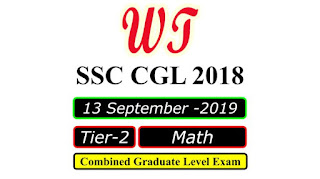Type Here to Get Search Results !

# Download SSC CGL 2018 Tier 2, Math Paper, 13 September 2019

Download SSC (Staff Selection Commission) CGL (Combined Graduate Level Examination) 2018 Tier 2 ,Math Paper Was Held on 13 September 2019.SSC CGL 2018 Tier 2 Math 13 Sep 2019 Paper PDF

Question :- 01
Two-third of the number of employees of a compony are males and the rest are females. If 3/8 of the male employees and 2/5 of the female employees are temporary employees and the total number of permanent employees is 740. then 7/15 of the total number of employees exceeds the number of temporary female employees by:
a.    400
b.    340
c.    308
d.    320

Question :- 04
What is the ratio of the third proportional to 0.4 and 0.8, to the mean proportional between 13.5 and 0.24?
a.    5 : 4
b.    7 : 8
c.    8 : 9
d.    9 : 10

Question :- 07
A sum lent out at simple interest amounts to Rs.6076 in 1 year and Rs.7504 in 4 years. The sum and the rate of interest p.a. are respectively:
a.    Rs.5,600 and 9%
b.    Rs.5,600 and 8.5%
c.    Rs.5,400 and 9%
d.    Rs.5,400 and 10%

Question :- 08
In ∆ABC , the medians AD, BE and CF meet at O. What is the ratio of the area of ∆ABD to the area of ∆AOE?
a.    2 : 1
b.    3 : 1
c.    5 : 2
d.    3 : 2

Question :- 11
5 years ago, the ratio of the age of A to that of B was 4 : 5. Five years hence, the ratio of the age of A to that of B will be 6 : 7. If, at present, C is 10 years younger than B, then what will be the ratio of the present age of A to that of C?
a.    3 : 2
b.    5 : 4
c.    4 : 3
d.    5 : 3

Question :- 16
In a circle, AB and DC are two chords. When AB and DC are produced, they meet at P. If PC = 5.6 cm, PB = 6.3 cm and AB = 7.7 cm, then the length of CD is:
a.    8.35 cm
b.    9 cm
c.    10.15 cm
d.    9.25 cm

Question :- 20
A spends 65% of his income. His income is increased by 20.1% and his expenditure is increased by 25%. His savings:
a.    Increase by 11%
b.    Increase by 5%
c.    Decrease by 5%
d.    Decrease by 11%

Question :- 21
The average weight of a certain number of students in a group is 72 kg. if 10 students having an average weight of 78 kg leave and 4 students having an average weight of 80 kg join the group, the average weight of the students in the group decreases by 0.7 kg, The number of students initially in the group is:
a.    56
b.    46
c.    44
d.    54

SSC CGL 2018 Tier 2 All Papers